• c语言 for循环嵌套(含答案)
2021-05-23 06:02:24

c语言 for循环的嵌套(含答案)

第 33、34 课时 for 循环的嵌套实验题一：1、下面程序的功能是计算:至 50 中是 7 的倍数的数值之和,请选择填空。D#include main() int i,sum= 0; for(i=1;i Bmain() int a,b,c,i; a=c=0; for(i=0;imain() int i; #imain() int x,i; for(i=1;imain()int i,b,k=0;for(i=1;i=0) k+;printf(“%d,%d“,k,b);A)3,-1 B )8,-1 C)3,0 D)8,-2实验题二：编程题：1、编写程序，输入从公元 1000 年至 2000 年所有闰年的年号。每输出 3 个年号换一行。判断公元年是否为闰年的条件是：(1) 公元年数如能被 4 整除，而不能被 100 整除，则是闰年；(2) 公元年数能被 400 整除也是闰年。#include#include main()int i,j=0; for(i=2000;i=3000;i+)if(i%4=0j+;if(j%10=0)printf(“n“);2、使用嵌套的循环结构在屏幕上打印下面的图形。* * * * *方法一：方法一：main()main() intint i,j,k;i,j,k;for(i=0;i15;i+)for(i=0;i15;i+)printf(“*“);printf(“*“);printf(“n“);printf(“n“);for(j=0;j4;j+)for(j=0;j4;j+)printf(“*printf(“* *n“);*n“);for(k=0;k15;k+)for(k=0;k15;k+)printf(“*“);printf(“*“);getch();getch(); 方法二：方法二：main() int i,m,j;for(i=1;i=6;i+)if(i=1|i=6)for(j=1;j=16;j+) printf(“*“); printf(“n“);elsefor(m=1;m=16;m+) if(m=1) printf(“*“); if(m=16)printf(“*n“); elseprintf(“ “);

更多相关内容
• C语言 for循环嵌套题(含解析和答案)第33、34课时 for循环嵌套实验题一：1、下面有关for循环的正确描述是: DA) for循环只能用于循环次数已经确定的情况B) for循环是先执行循环体语句,后判断表达式C) 在for循环中,...

C语言 for循环的嵌套题(含解析和答案)

第33、34课时 for循环的嵌套

实验题一：

1、下面有关for循环的正确描述是: D

A) for循环只能用于循环次数已经确定的情况

B) for循环是先执行循环体语句,后判断表达式

C) 在for循环中,不能用break语句跳出循环体

D) for循环的循环体语句中, 可以包含多条语句,但必须用花括号括起来

2、对for(表达式1;;表达式3)可理解为:B

A) for(表达式1; 0;表达式3)

B) for(表达式1;1;表达式3)

C) for(表达式1;表达式1;表达式3)

D) for(表达式1;表达式3;表达式3)

3、若i为整型变量,则以下循环执行次数是:B

for (i=2;2==0;) printf("%d",i-- );

A)无限次 B) 0次 C) 1 次 D) 2次

4、以下for循环的执行次数是:C

for (x=0,y=0; (y=123)&&(x<4); x++) ;

A)是无限循环 B)循环次数不定 C)执行4次 D)执行3次

解析：第一次：;y=123&&0<4成立x++(x=1；第二次：(y=123)&&(1<4)成立，x++(x=2

第三次：(y=123)&&(2<4)成立，x++(x=3 第四次：(y=123)&&(3<4)成立，x++(x=4

第五次：(y=123)&&(4<4)不成立，退出循环。

5、以下不是无限循环的语句为:A

A) for (y=0,x=1;x > ++y;x =i++) i=x ;

B) for (;1; x++=i);

C) while (1) {x ++;}

D) for(i=10;1 ;i--) sum+=i;

6、下面程序段的运行结果是:C

for (y=1;y<10;)

y=((x=3* y,x+1),x-1);

printf ("x=%d,y=%d",x,y);

A)x=27,y=27 B)x=12,y=13 C)x=15,y=14 D)x=y=27

第一次：1<10为真，x=3,x+1=3+1=4, y=(4,x-1) y=(4,2)=2

第二次： 2<10为真， x=3*2=6,7 y=(7,x-1)=(7,5) y=5

第三次：5<10为真，x=3*5=15 16 y=(16,x-1)=(16,14) y=14

第四次： 14<10为假，

7、下面程序段的运行结果是

for(x=3;x<6;x++)

printf((x %2)?("**%d"):(" ##%d\n"),x);

解析：第一次：3<6为真，3%2=1 **3 x++(x=4

第二次：4<6为真，4%2=0 ##4(换行) x++(x=5

第三次：5< 6为真，5%2=1 **5 x++(x=6

第四次：6<6为假，结束循环

A)**3 B )##3 C)##3 D)**3##4

##4 **4 **4##5 **5

**5 ##5

8、下列程序段不是死循环的是

A) int i=100;

whiLe (1)

{i=i%100+1;

if (i>100) break;

}

B) for(;1;);

C) int k=0;

do{++k;}

while (k> =0) ;

D) int s=36;

while (36) ; --s;

9、执行语句for(i=1;i++<4;) ;后变量i的值是

A)3 B )4 C)5 D)不定

实验题二：编程题

1、编写程序，求1-3+5-7+…-99+101的值。

(1+5+9+13+17+…+101)-(3+7+11+15+…+99)

方法一：

main()

{

int i,j,sum1=0,sum2=0,sum;

for(i=1;i<=101;i+=4)

sum1+=i;

for(j=3;j<=99;j+=4)

sum2+=j;

sum=sum1-sum2;

printf(“1-3+5-7+…-99+101=%d”,sum);

}

方法二：main() { ???int?i,sum=0,s=1; ???for(i=1;?i<=101;i

展开全文• for循环语句的特点，详细介绍哈。

大家好，相信刚学C语言的小白们，觉得for循环是比较好用的，但是有时候写for循环语句的程序时，写出来的代码结果，和自己想要的不是一个结果，认为自己写的是对的，但其实是错的，我刚学习的时候也是，下面我分享一下两个程序一个是单循环，一个是for的循环嵌套，是经典易错的误区一起来看看吧。

首先来了解一下for循环语句；如果了解的小伙伴可以直接跳过，看例题；

我们都知道for循环分为三个部分为：

for（表达式1；表达式2；表达式3）

表达式1：可以用来赋初值。可以写多个语句，但是这多个语句要用逗号分开。（也可以不写,根据需求来进行编写）

表达式2：判断条件是否成立，如果成立，进入循环体；（同样也可以省略不写，如果不写就默认是1，也就是恒成立的,也就是死循环。如果要跳出循环，需要在循环体中编写break语句）

表达式3：一般用来定义循环变量的自增或自减；（同样也可以省略不写；这个循环变量可以在循环体中进行设置）

所以我们可以这三个语句我们都可以省略不写。但是切记用来分隔三个表达式的分号‘；’不可不写。

下面看例题：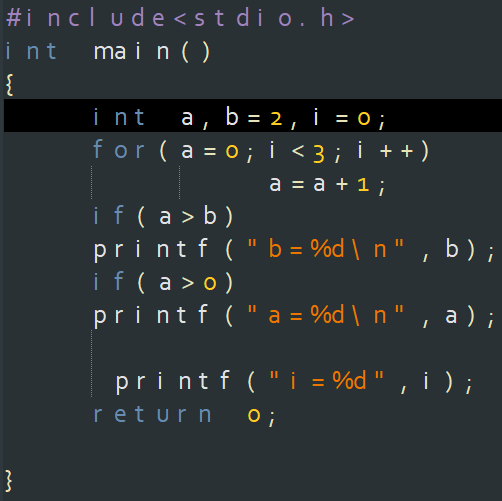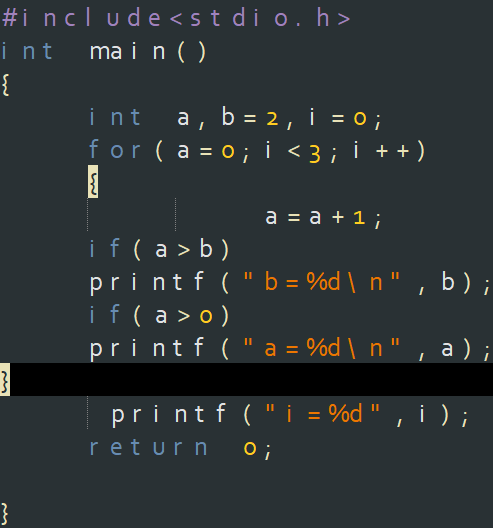其实乍一看这两程序是一样的，但仔细看，其是是有很大的不同的。第一个for的循环中有‘{}’，而第二个没有，那么这两个程序的结果，就是不一样的，而且，如果不注意这点，以后编写调用函数利用for循环，那么结果可是截然不同的，这两个结果分别为: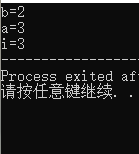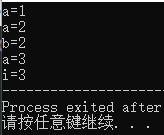因为如果不加花括号，和其他循环体一样只会判断它下面的第一条语句是否为真，如果为真，则执行完这条语句后返回循环首部进行判断是否再次进入循环，并不是往下进行下一条语句。直到循环结束，然后执行下面的语句，如果不为真，则直接返回循环首部，进行第二次判定是否进入循环，直到不满足条件，循环结束，跳出循环。执行下面的语句。for语句和它下面的第一条语句构成一个循环体结构，如果有花括号，就是for语句和花括号里的内容构成循环体结构，就会依次执行花括号里面的语句，进行循环。（搭配一个小草图）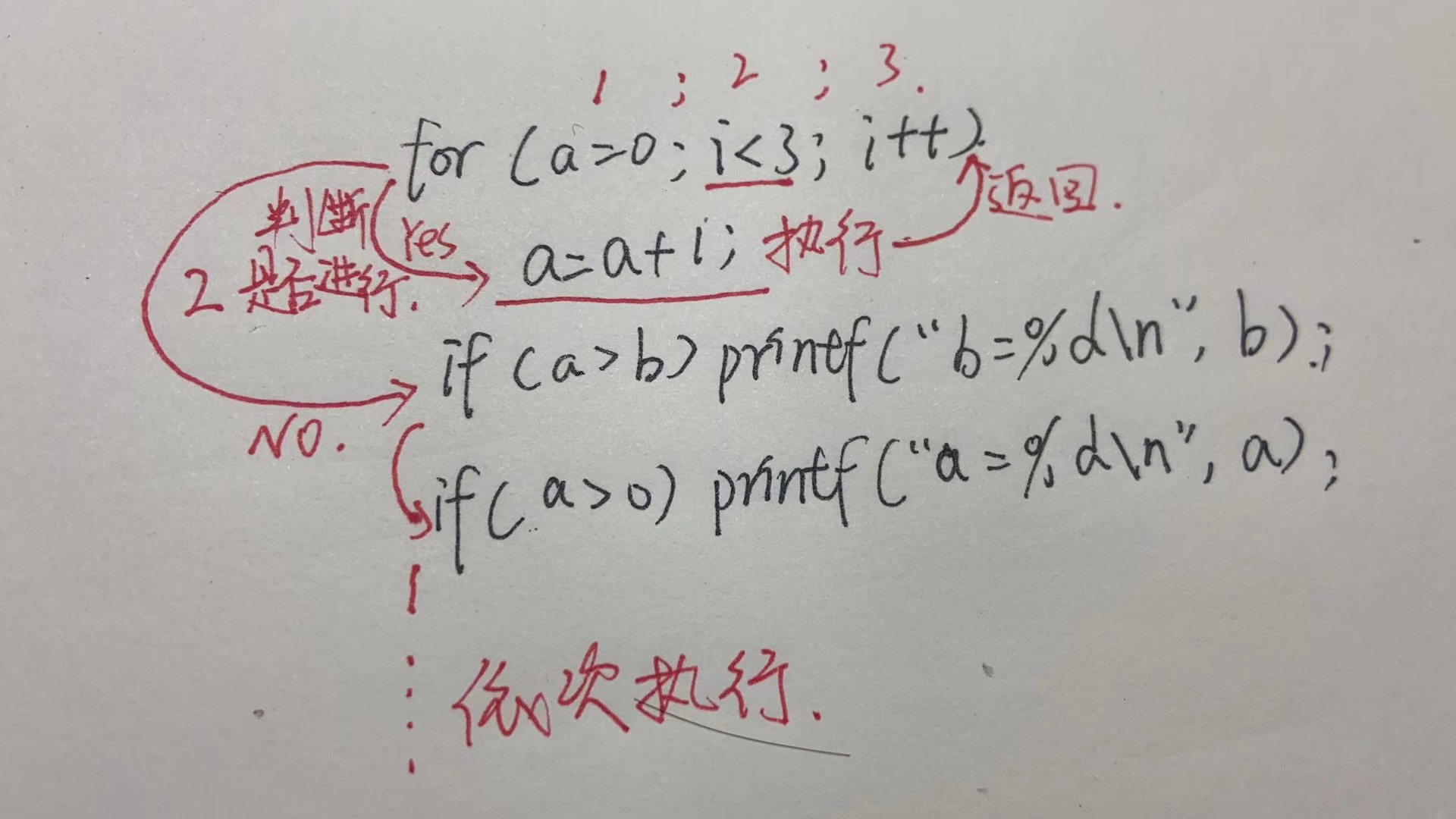第二个例句for循环的嵌套：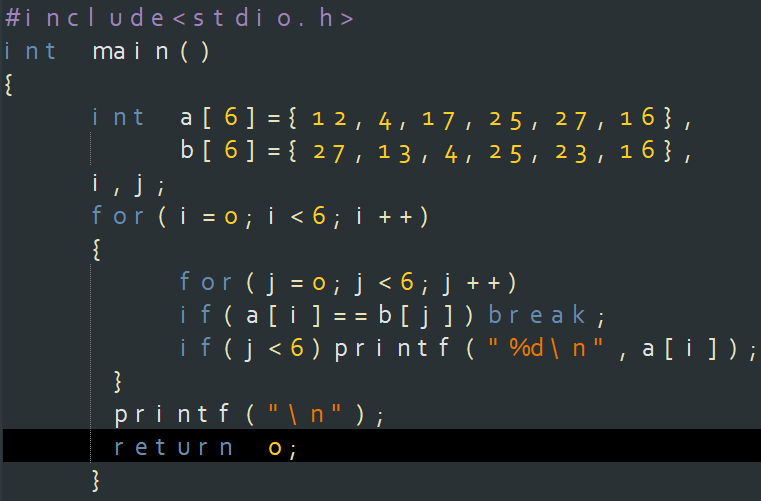如果上面的你已经掌握了，那么来试着想想这道程序的结果吧，我把结果留在最后，理解的朋友可以直接跳过看结果，不理解的朋友可以看看讲解。

这个程序和上面的程序，其实是一样的只不过是进行了一次嵌套，里面的for语句叫内循环，外面的for语句叫外循环。外循环执行一次，内循环执行一轮，全部执行完之后，返回外循环，重新判断，是否第二次进入内循环。

首先进入外循环，i的值为0，然后进入第二层循环，j的初值为0；进入内循环，判断a[i]是否等于b[j],如果等于，跳出第二层循环执行第一层循环i++；如果你是这么理解的，那么说明你还对上面的不太了解在这里其实并不是跳出内循环，然后去执行外循环语句，而是依次执行下面的语句，直到碰到花括号，结束本次花括号里的内容，返回外循环；因为上面说过for循环中没有花括号的话，只执行它下面的第一条语句；如果a[i]的值不等于b[j]不成立，则返回第内循环首部进行j++，然后判断是否再次进入内循环，直到循环结束如果都不满足下面的语句，那么循环结束，自动返回外循环首部，它下面的换行是不会执行的，直到外循环结束，才会执行。同理如果内循环中有花括号'{}',那么就会依次执行了。不论第一条语句是否成立，都会依次往下进行。（再次搭配一个小草图）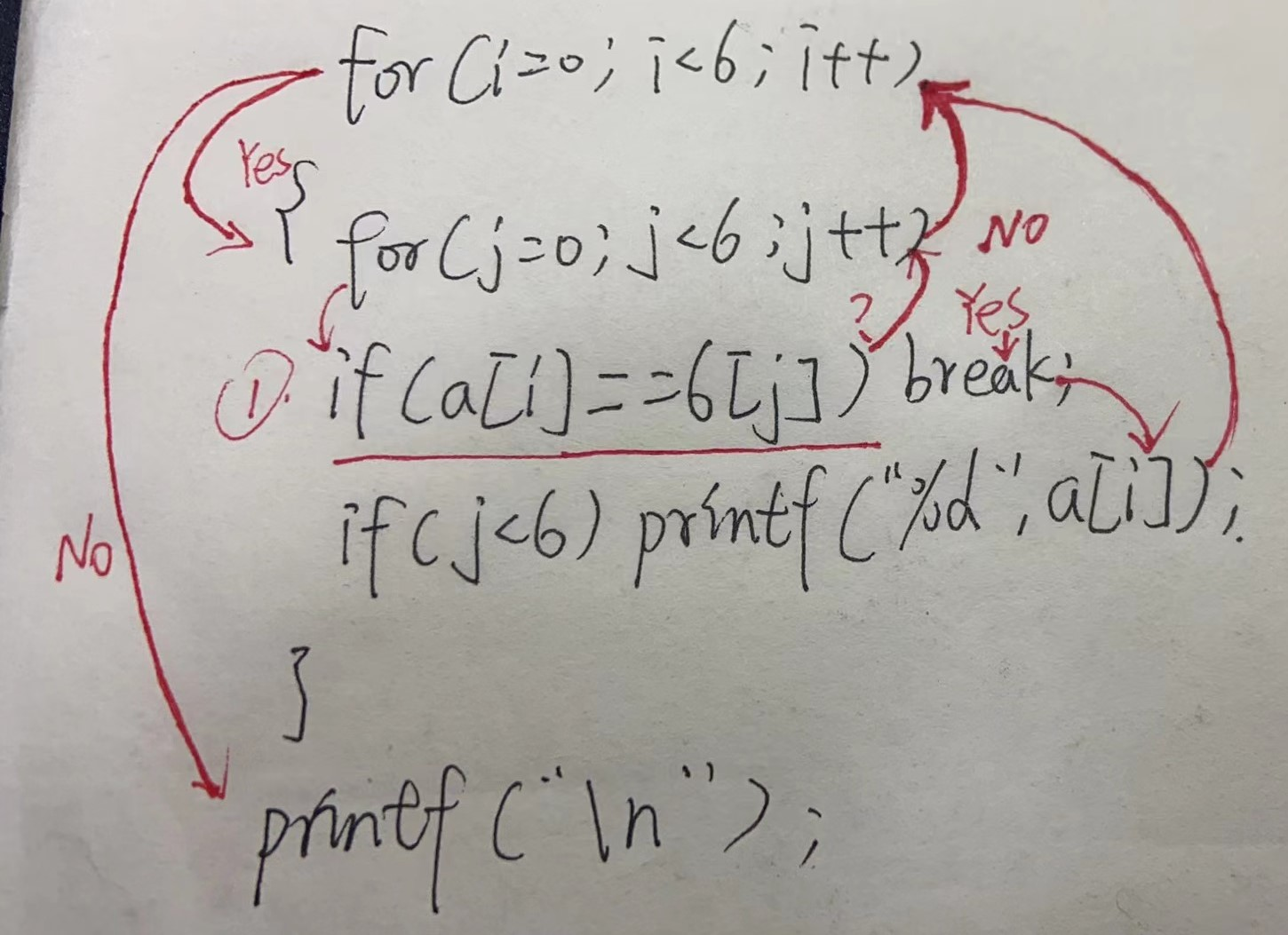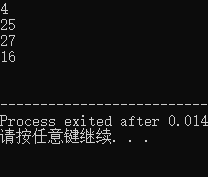好了以上就是本篇，内容，希望对困惑的小伙伴有帮助。如果哪里有不对的，请立刻指正，不要让我错下去。（手动滑稽） 。

展开全文• #include<stdio.h> int main() { int i=0; int j=0; for(i=1;i<=4;i++){ for(j=1;j<=5;j++){ printf("%d ",i*j); } printf("\n"); } return 0; }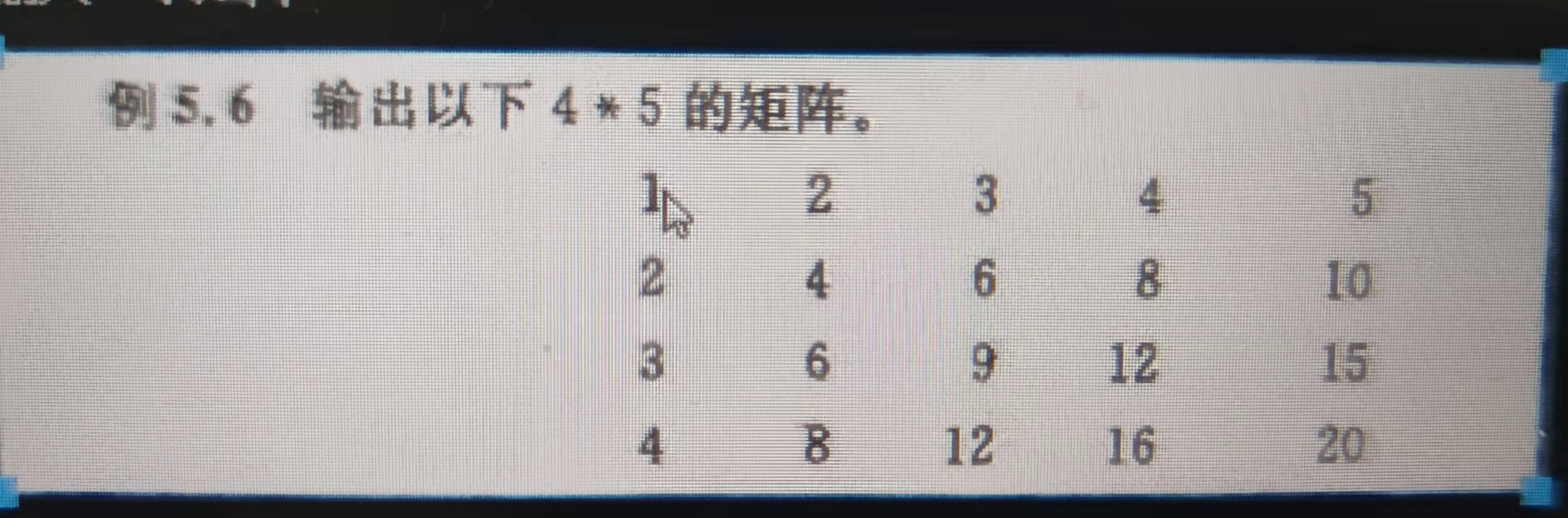#include<stdio.h>
int main()
{

int i=0;
int j=0;

for(i=1;i<=4;i++){

for(j=1;j<=5;j++){
printf("%d   ",i*j);
}

printf("\n");
}
return 0;
}


展开全文开发语言
• C语言 for循环嵌套(含答案).doc第33、34课时 for循环嵌套实验题一：1、下面程序的功能是计算:至50中是7的倍数的数值之和,请选择填空。D#includemain(){int i,sum= 0;for(i=1;i<=50;i++)if() sum+=i;printf...
• 2013-04-01我想问一下图中C语言的两个分支语句有什么区别，好像一样，可是书中把一个分类为if的嵌套，一个分为else if语句！为什么不一样两个语句，else-if语句：意思是有多个条件语句，根据不同的条件语句得到的...
• 循环嵌套的定义：一个循环体内又包含另一个完整的循环结构。内嵌的循环中还可以嵌套循环，这就是多层循环。 可以在三个基本循环中，随意选择随意组合。 循环方式：（假设共两层循环）在外层循环执行一次之后，进入内...
• 金字塔核心思想很简单，就是根据行列与字母，或者其他字符出现次数之间的关系建立测试条件，使用嵌套循环，即可解答。再此，列入经典程序。 /* A ABA ABCBA ABCDCBA ABCDEDCBA */ #include <stdio.h> #...算法
• 1、例题 1、1不从循环跳出 #include <stdio.h> #include <stdlib.h> int main() { int x; scanf("%d",&x); int one; int two; int five; for(one=1;one<x*10;one++){ for(two=1;two...开发语言 后端
• 原标题：C语言从入门到精通：嵌套... 语法在C语言嵌套for语句循环的语法如下：for ( init; condition; increment ){ for ( init; condition; increment ) { statement(s); } statement(s);}在C编程语言中嵌套while...
• c语言for循环练习题精品文档2016全新精品资料-全新公文范文-全程指导写作 –独家原创 PAGE1 / NUMPAGES1c语言for循环练习题目的和要求：1、 掌握循环结构程序设计的方法2、 掌握简单循环程序设计方法3、 掌握for循环...
• 需要知道的是，两个for循环嵌套的时候，都是里面的for循环结束以后才会开始外面一层for循环 情况一： a = ['a','b'] b = ['1','2'] ​ c = [] #因为c在所有循环外面，所以每次循环到append的时候都会把添加元素 ...python list dict
• 计算5的阶乘 利用for循环 然后在含有main（）的源程序中引入后调用该方法 #include<stdio.h>...嵌套for循环的事例 计算s=1+（1+2）+（1+2+3）+。。。。+（1+2+。。+20） #include<stdio.h>
• for循环：for( ; ; )里面是bai3个语句，两个分号。第一个语句是开始前执行，第二个语句是判断真假，如果真，就...关于嵌套for循环理解例题：include include int main(){for(int i=0;i<5;i++){//第一个for语句...
• ## C语言循环经典例题

千次阅读 多人点赞 2020-07-26 17:14:53
判断一个数是否为素数 #include <stdio.h> int main() { int x; scanf("%d", &x); int i;... for ( i=2; i<x; i++) { if ( x % i == 0) { isprime = 0; break; } } if ( ispri
• 又因为是查找题，数量很大，把这些数列出来肯定要用的循环语句，由此可知，for循环嵌套if判断语句的嵌套。因为需要一个四位数，而且要知道每位数的数字，所以会用到以下方法。 看成一个四位数也就是从1000到9编程语言 c算法 算法
• 第 5 章 循环结构程序设计 5.1 基本知识点 while 语句的使用格式和注意事项 do-while 语句的使用格式和注意事项 for 语句的使用格式和注意事项 break 和 continue 语句在循环语句中的应用 循环结构的嵌套 使用 goto ...
• for循环嵌套里怎幺用if语句控制外循环?修改如下int Su(int x){int i,j;for(i=x;i>=2;i--){for(j=2;j<=i>=i>{if(i%j==0) {break;} //若能整除任何一个数，说明非素数，跳出内循环，检查下一个i}if(j>i...
• C语言第三课 for循环例子.doc下载提示(请认真阅读)1.请仔细阅读文档，确保文档完整性，对于不预览、不比对内容而直接下载带来的问题本站不予受理。2.下载的文档，不会出现我们的网址水印。3、该文档所得收入(下载+...
• 在使用循环嵌套打印多行数据时，由于打印方式是从上向下、从左向右。因此，通常行数使用外循环，行内使用内循环。对于上图中每一行中元素的个数是一定的，这种独立的，这种实现就简单了： 思路：使用两个变量：i ...
• 三种循环(while循环、do-while循环和for循环)可以互相嵌套。下面几种都是合法的形式：几种循环的比较(1)四种循环都可以用来处理同一问题，一般情况下它们可以互相代替。但一般不提倡用goto型循环。(2)在while循环和...
• 使用嵌套循环产生下列图案\$首先确定行数为i=5，因此外套循环的终止条件是i到达6时退出循环；由列数为5并且每一列元素数逐渐递增比变化可知，内层循环的判定条件j受外层循环的影响，由图可知第一行一个...
• 循环语句是在C语言经常遇到的一种控制语句。在实际应用中的许多问题，都会涉及重复执行一些操作，如级数求和、穷举或迭代求解等。 C语言提供for、while、do-while三...for循环的一般形式为： for（表达式1；表达式2；表
• 一个十分经典的 for循环嵌套 例题 问题：计算出自然数 SIX 和 NINE ( 它们满足的条件是 SIX + SIX + SIX = NINE +NINE ) 的个数cnt。其中的S、I、X、N、E各代表一个十进制数字，S>0，N>0。 （一）陷阱：我...程序人生 开发语言
• 精心整理 第 5 章循环结构程序设计 5.1 基本知识点 while 语句的使用格式和注意事项 do-while 语句的使用格式和注意事项 for 语句的使用格式和注意事项 break 和 continue 语句在循环语句中的应用 循环结构的嵌套 ...
• 图形的样式千奇百怪，但是大部分都是万变不离其宗，所以说，要想彻底掌握这些，应该做到学以致用。例： 此题可以看作是一个上下对称的图形不妨先来看上面部分如何打印。 注：为了方便讲解，特地将...for(i=1;i&l开发语言 后端
• 满意答案#include#includevoid SanJiaoBo(){int x...for(y= 1; y <= 5; y++){for(x= 1; x <= 50; x++){if( y == x-5 ||y == x-13 ||y == x-21 ||y == x-29 ||y == x-37 ||y == -x+7 ||y == -x+15 ||y == -x+23...
• 循环经典题目 空心金字塔 public class Detail{ public static void main(String[] args){ /* 打印矩形 ***** ***** ***** ***** 1、打印出一半的金字塔 * ** *** **** ***** 2、...java 强化学习...